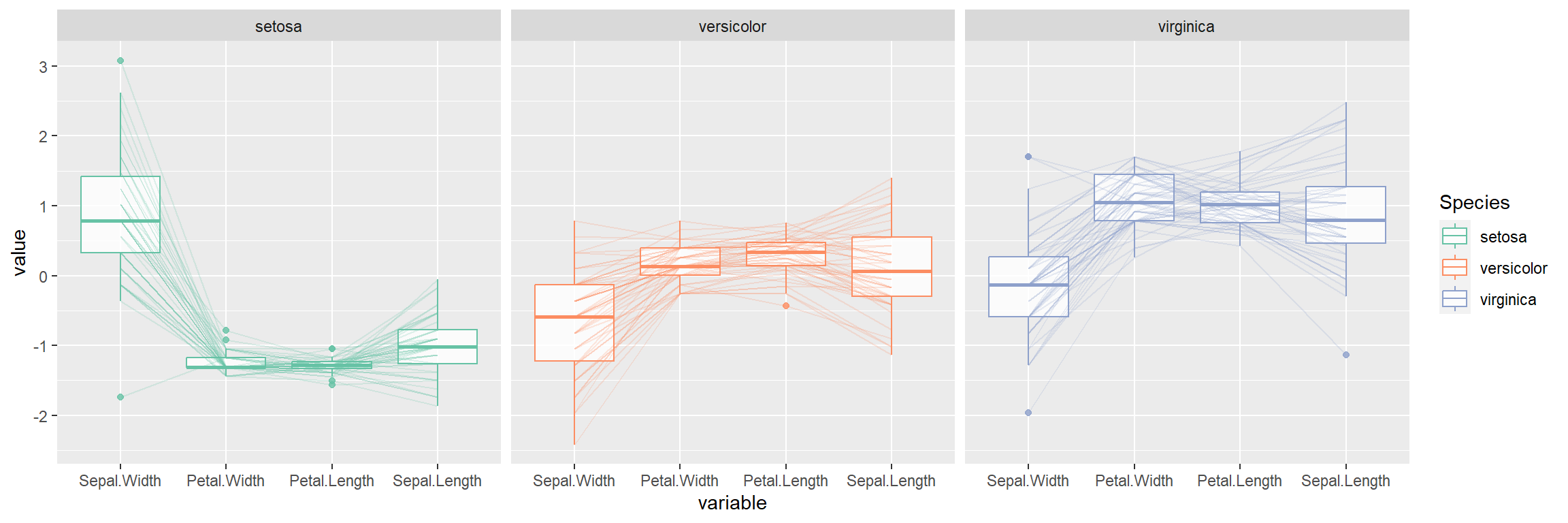# Parallel coordinates in ggplot2 with ggparcoord

Barret Schloerke

## Sample data set

In the examples below we are going to use the `iris` data set, which consists on different measures about the length and width of the petals and the sepals of flowers of different species.

``iris``

## Most basic parallel coordinate plot with `GGally`

The `ggparcoord` function from `GGally` packages allows creating parallel coordinate plots based on ggplot2. To create a basic plot pass the data frame to the function.

``````# install.packages("GGally")
library(GGally)

ggparcoord(data = iris)``````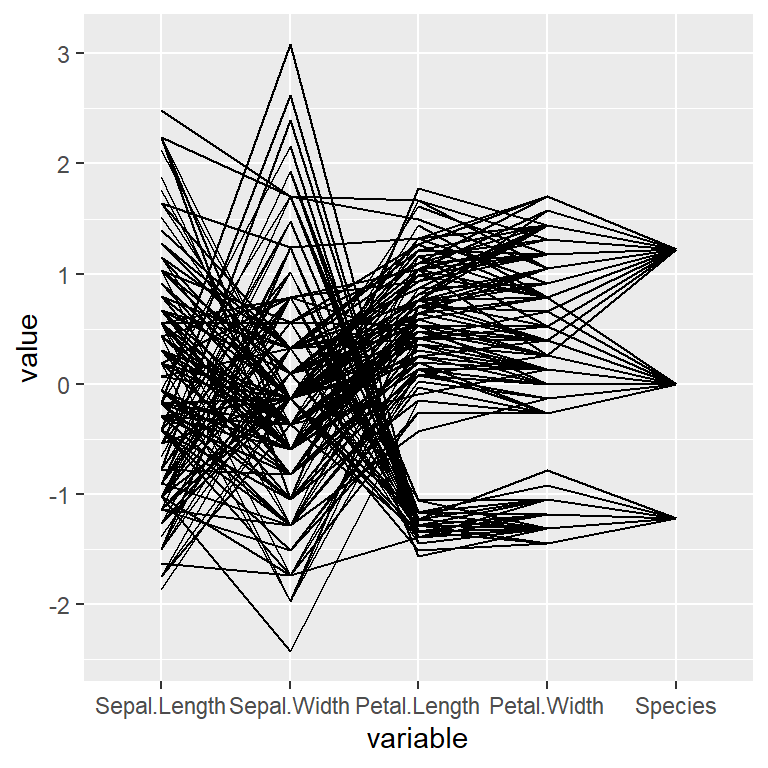Selecting columns

If you want to select only some columns to be displayed on the X-axis pass a vector of variables to the `columns` argument.

``````# install.packages("GGally")
library(GGally)

ggparcoord(data = iris,
columns = 1:4)``````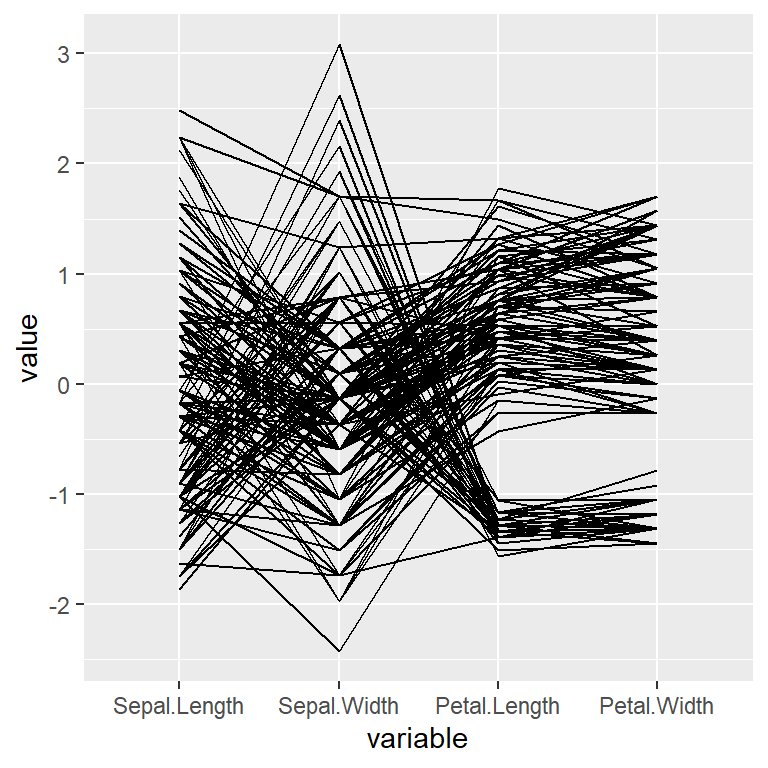Transparency of the lines

The transparency of the lines can be controlled with `alphaLines`. If there are many lines it is recommended to decrease the level of transparency.

``````# install.packages("GGally")
library(GGally)

ggparcoord(data = iris,
columns = 1:4,
alphaLines = 0.2)``````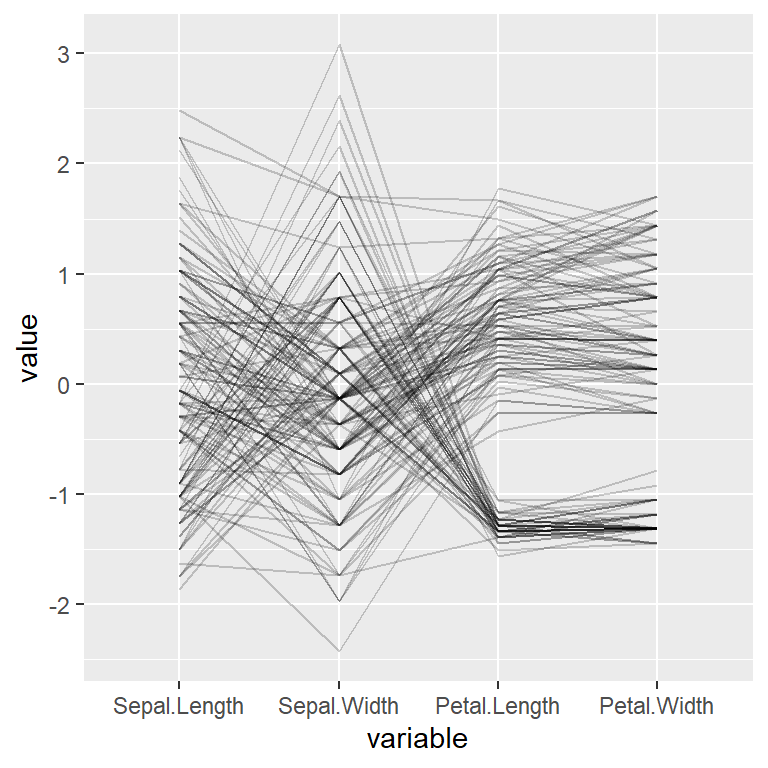Color by group

If the data set contains a categorical variable representing groups you can pass it to the `groupColumn` argument to colorize the lines by group.

``````# install.packages("GGally")
library(GGally)

ggparcoord(data = iris,
columns = 1:4,
groupColumn = "Species")``````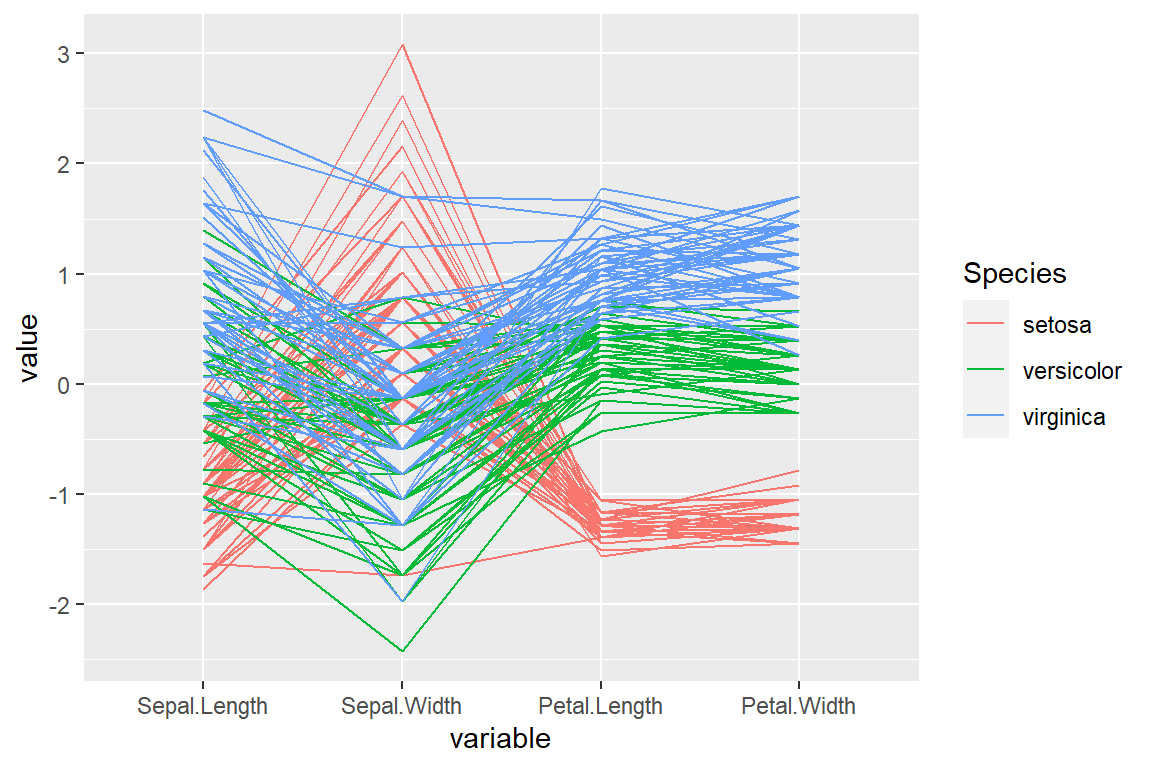Color customization

As the `ggparcoord` is based on ggplot you can change the color of the lines with `scale_color_manual` or `scale_color_brewer`, for instance.

``````# install.packages("GGally")
library(GGally)

ggparcoord(data = iris,
columns = 1:4,
groupColumn = "Species") +
scale_color_brewer(palette = "Set2")``````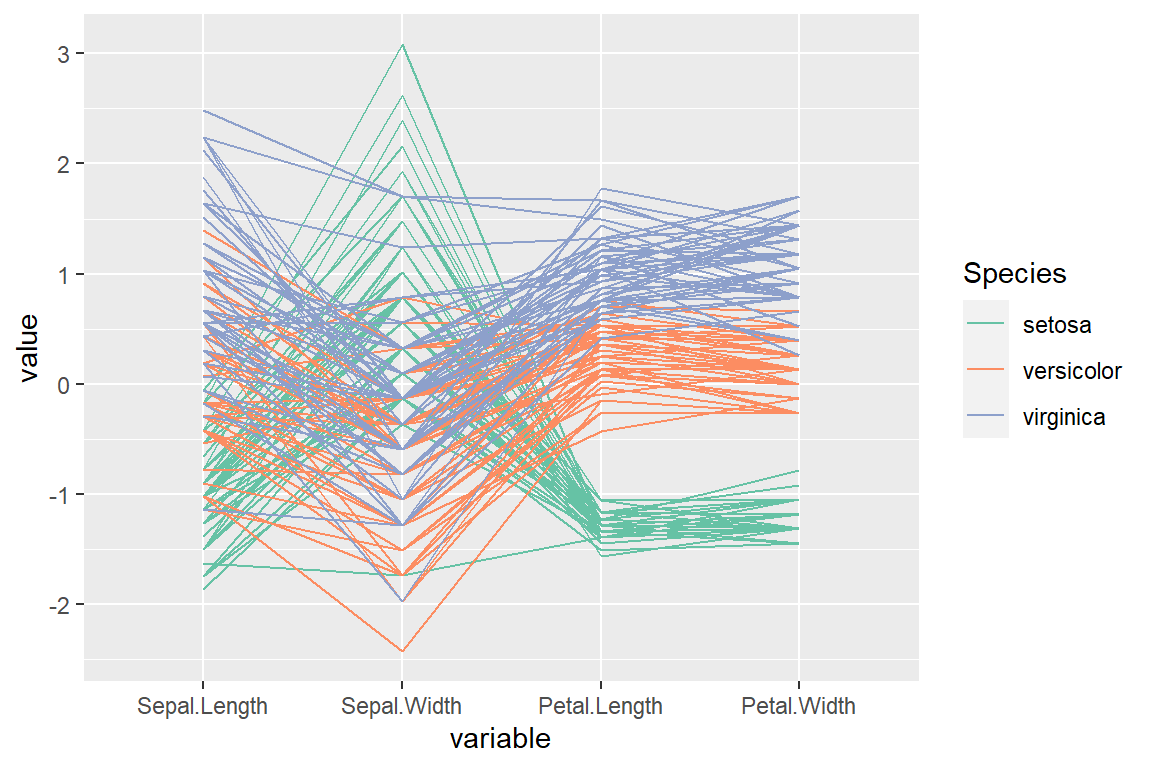The function provides a logical argument named `showPoints` which can be set to `TRUE` to plot the points.

``````# install.packages("GGally")
library(GGally)

ggparcoord(data = iris,
columns = 1:4,
groupColumn = "Species",
showPoints = TRUE) +
scale_color_brewer(palette = "Set2")``````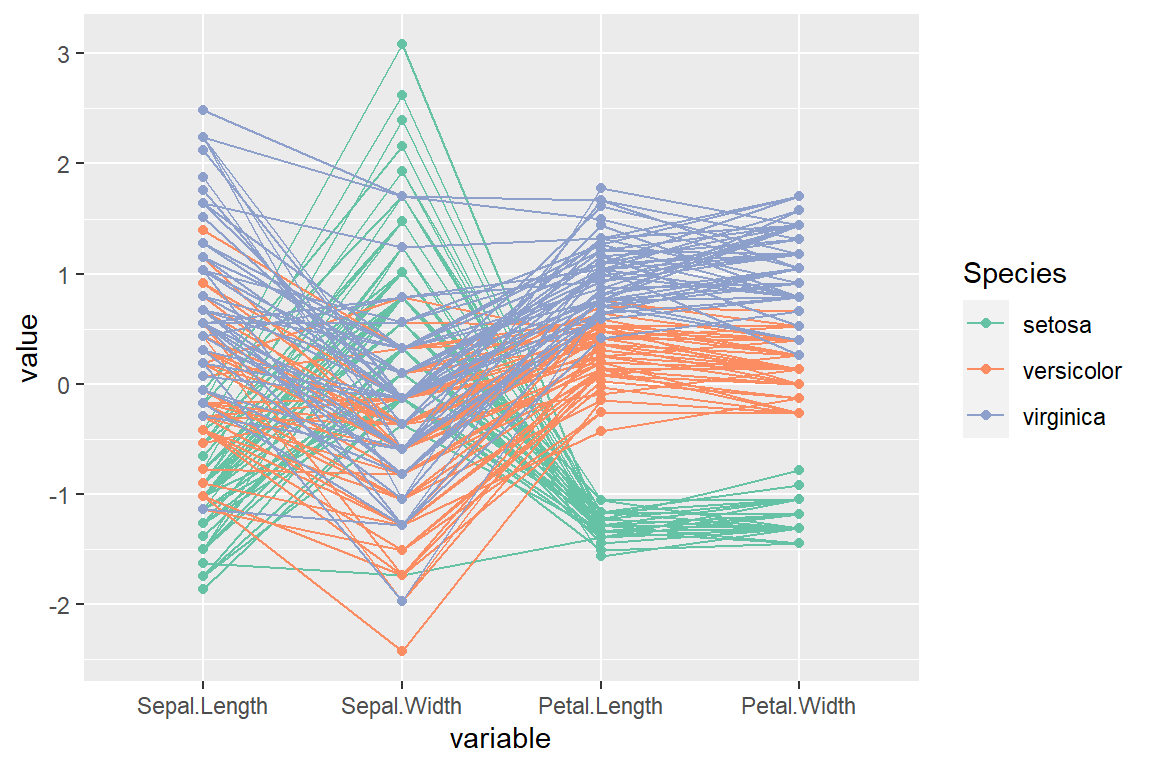Spline interpolation

The lines can be smoothed via spline interpolation setting `splineFactor = TRUE`.

``````# install.packages("GGally")
library(GGally)

ggparcoord(data = iris,
columns = 1:4,
groupColumn = "Species",
splineFactor = TRUE) +
scale_color_brewer(palette = "Set2")``````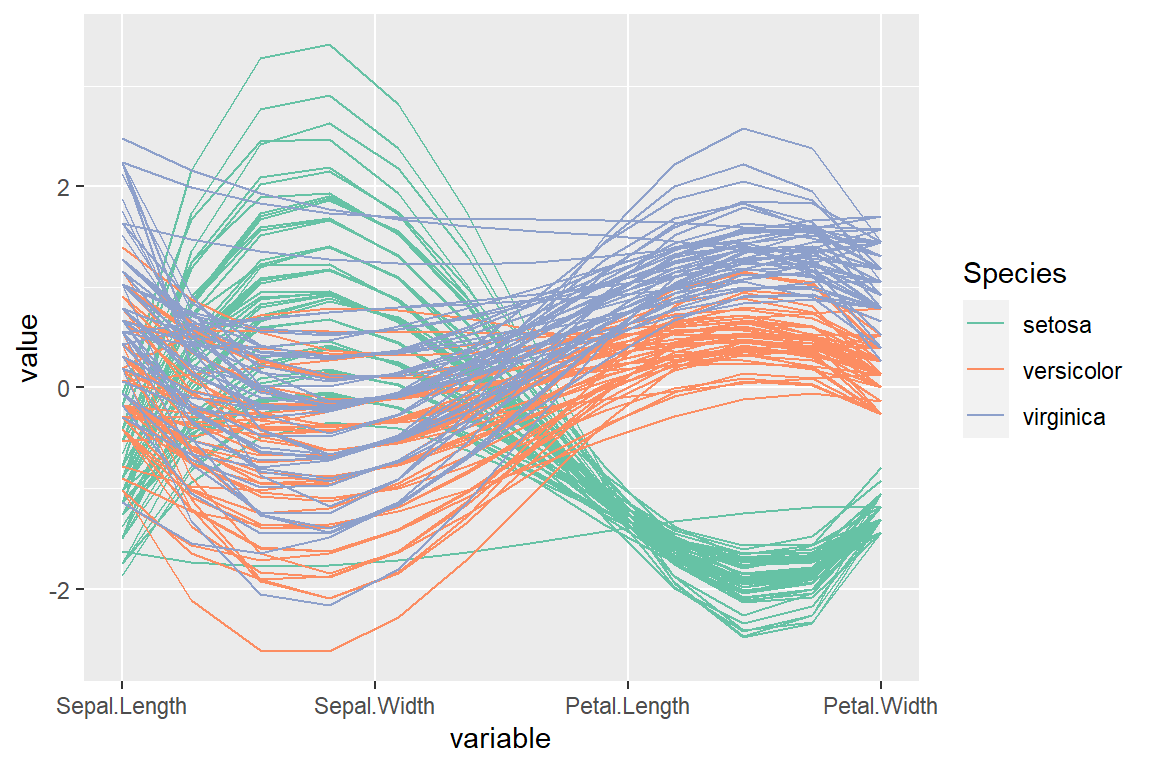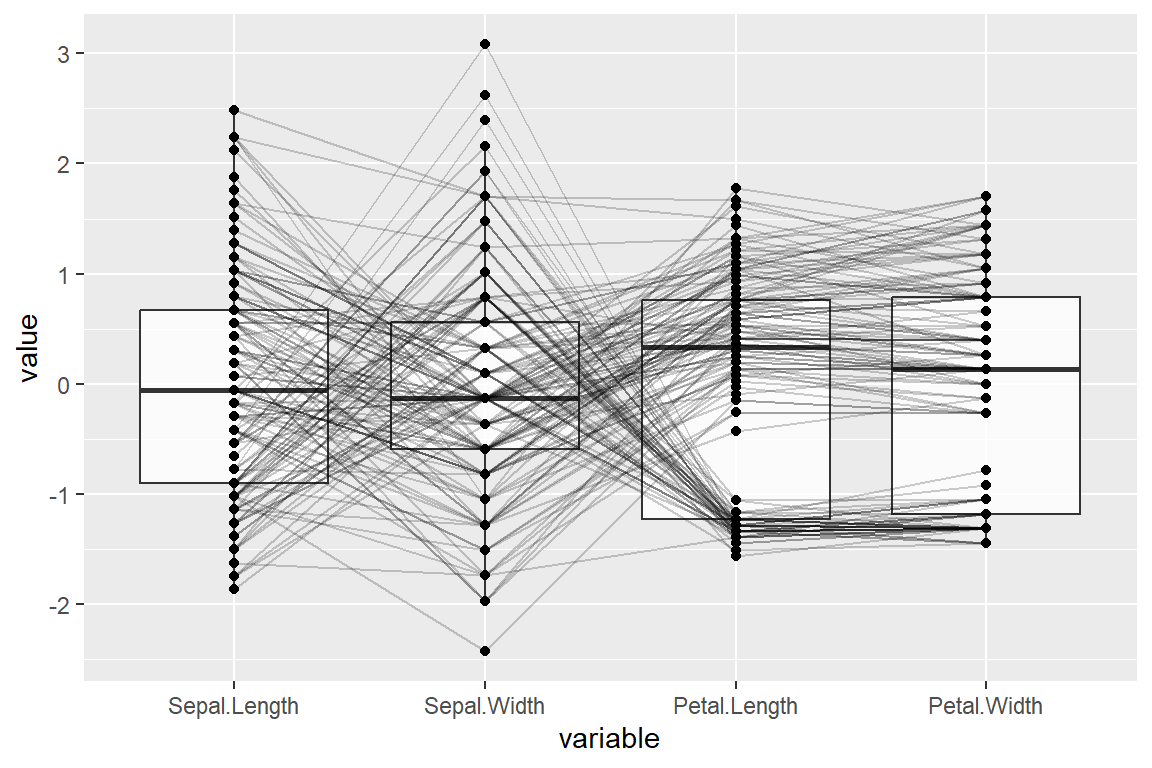It is possible to add box plots for each variable setting `boxplot = TRUE`.

``````# install.packages("GGally")
library(GGally)

ggparcoord(data = iris,
columns = 1:4,
alphaLines = 0.2,
showPoints = TRUE,
boxplot = TRUE)``````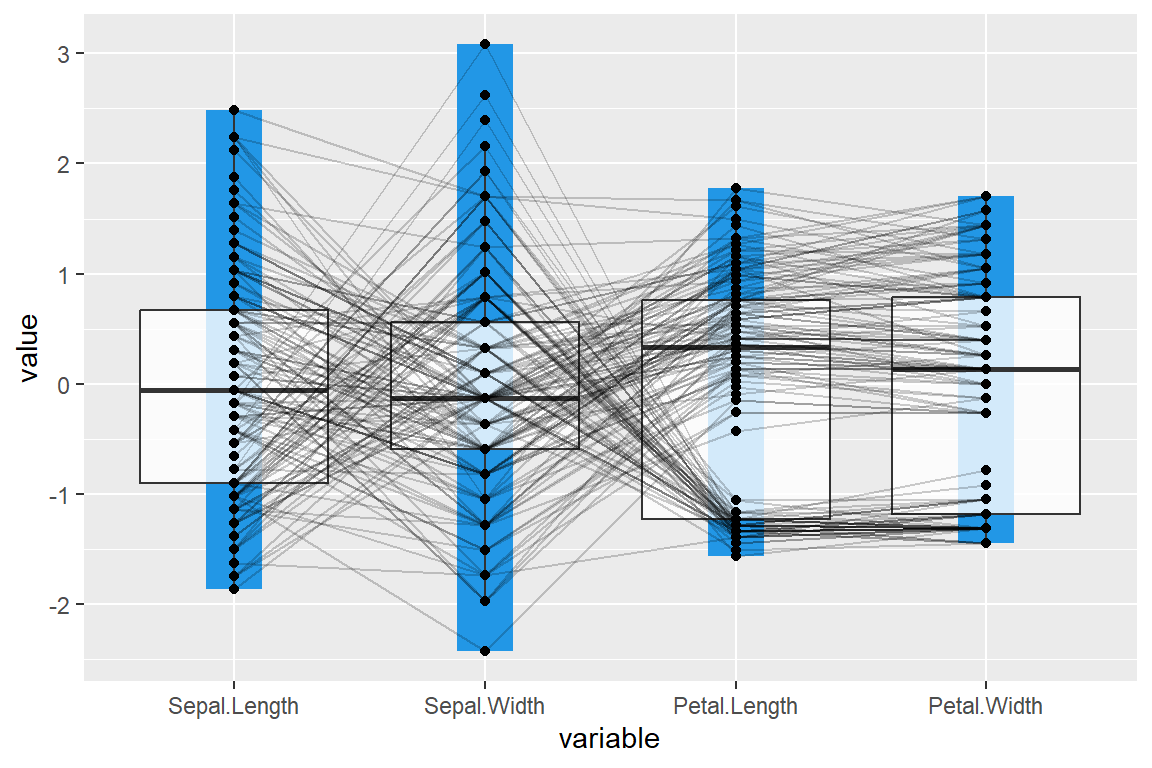You can also add a box from the maximum to the minimun for each variable.

``````# install.packages("GGally")
library(GGally)

ggparcoord(data = iris,
columns = 1:4,
alphaLines = 0.2,
showPoints = TRUE,
boxplot = TRUE,

## Scaling methods

The function provides several scaling methods that can be selected with the `scale` argument. The default method is `"std"` and the other possible options are displayed below. Type `?ggparcoord` for additional information.

“robust” scale

``````# install.packages("GGally")
library(GGally)

ggparcoord(data = iris,
columns = 1:4,
groupColumn = "Species",
scale = "robust") +
scale_color_brewer(palette = "Set2")``````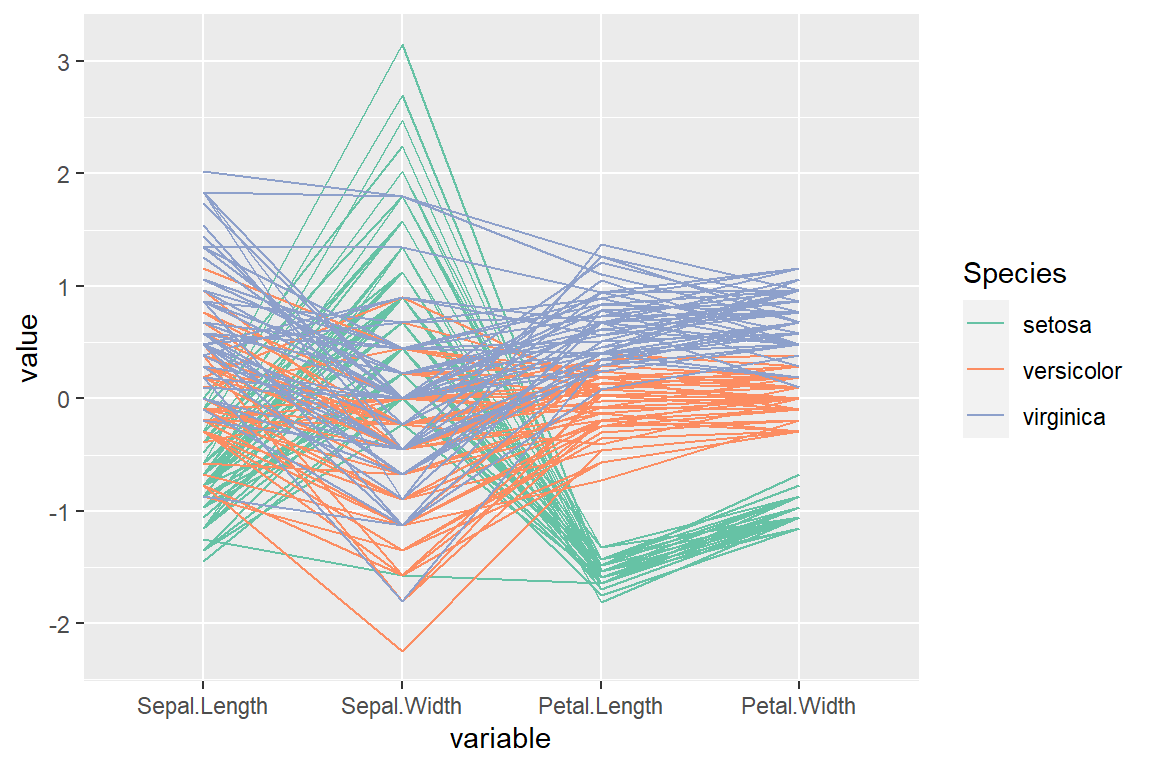“uniminmax” scale

``````# install.packages("GGally")
library(GGally)

ggparcoord(data = iris,
columns = 1:4,
groupColumn = "Species",
scale = "uniminmax") +
scale_color_brewer(palette = "Set2")``````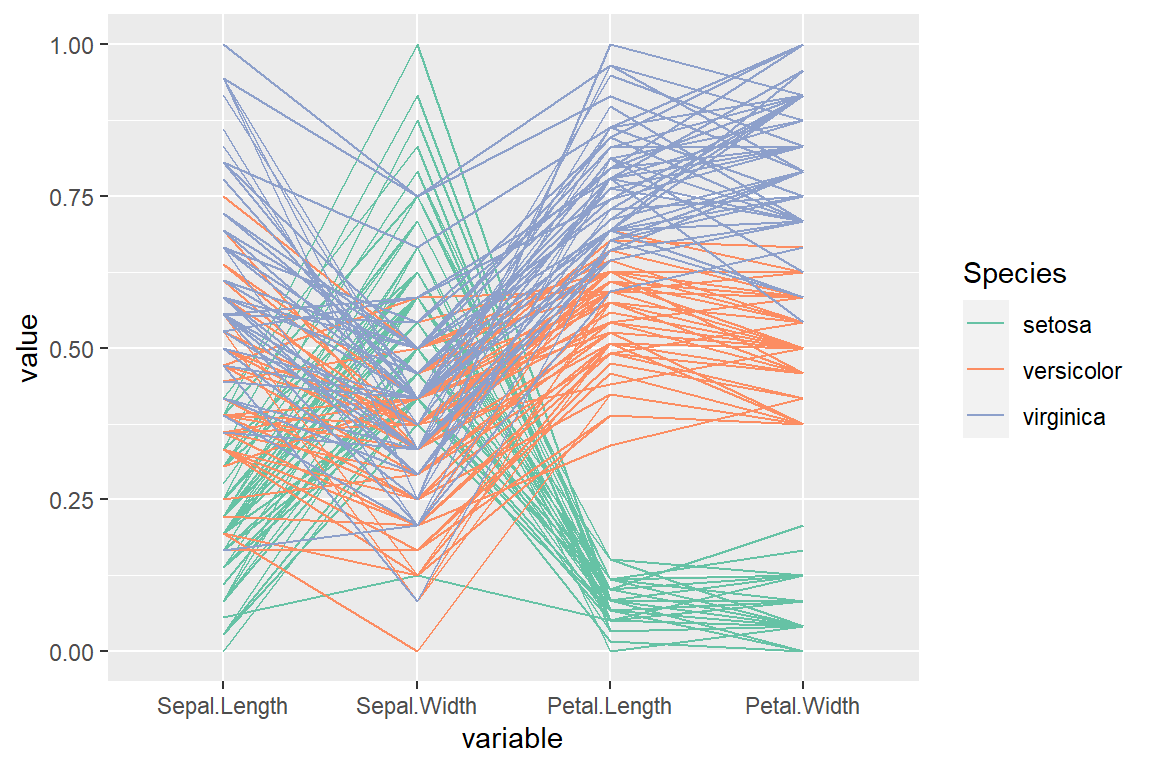“globalminmax” scale (no scaling)

``````# install.packages("GGally")
library(GGally)

ggparcoord(data = iris,
columns = 1:4,
groupColumn = "Species",
scale = "globalminmax") +
scale_color_brewer(palette = "Set2")``````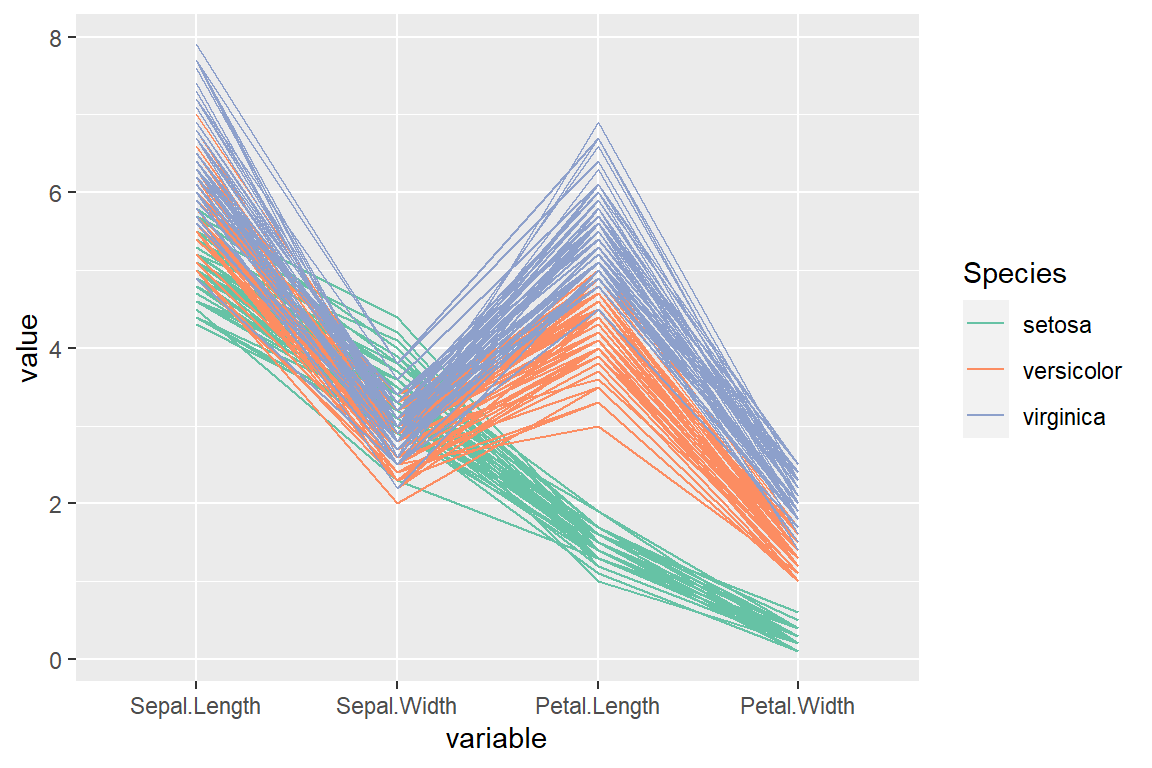“center” scale

``````# install.packages("GGally")
library(GGally)

ggparcoord(data = iris,
columns = 1:4,
groupColumn = "Species",
scale = "center") +
scale_color_brewer(palette = "Set2")``````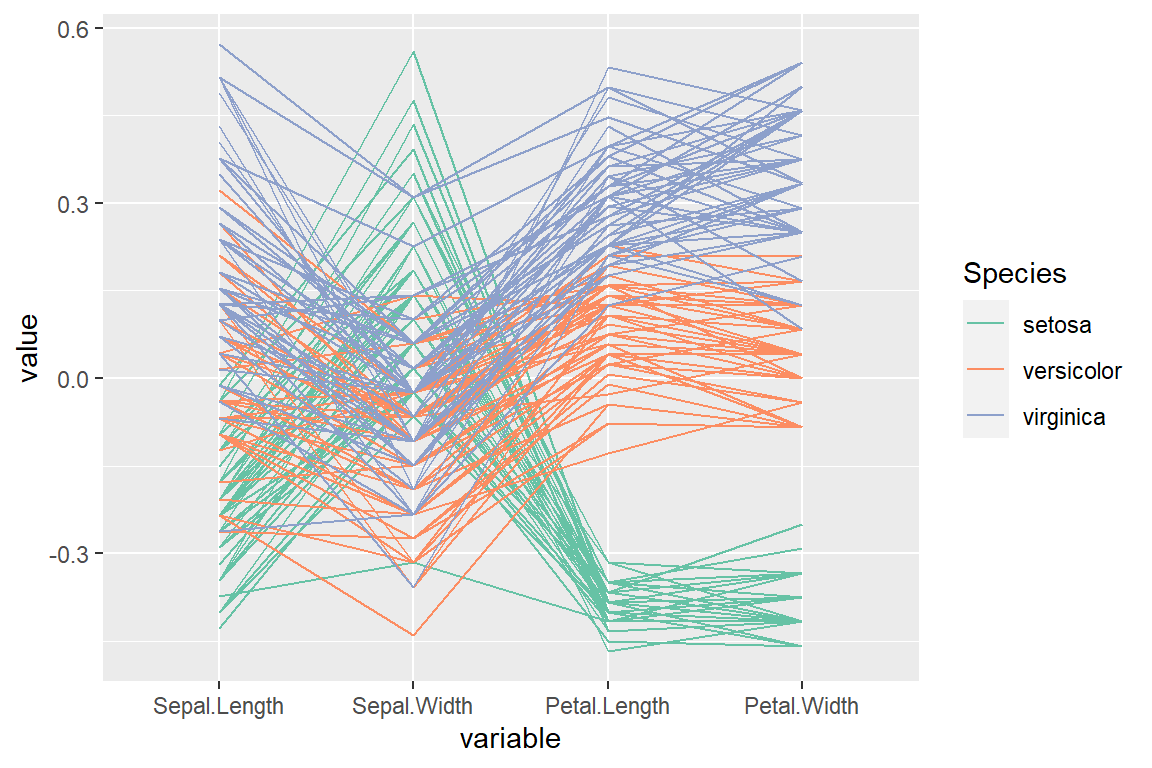“centerObs” scale

``````# install.packages("GGally")
library(GGally)

ggparcoord(data = iris,
columns = 1:4,
groupColumn = "Species",
scale = "centerObs") +
scale_color_brewer(palette = "Set2")``````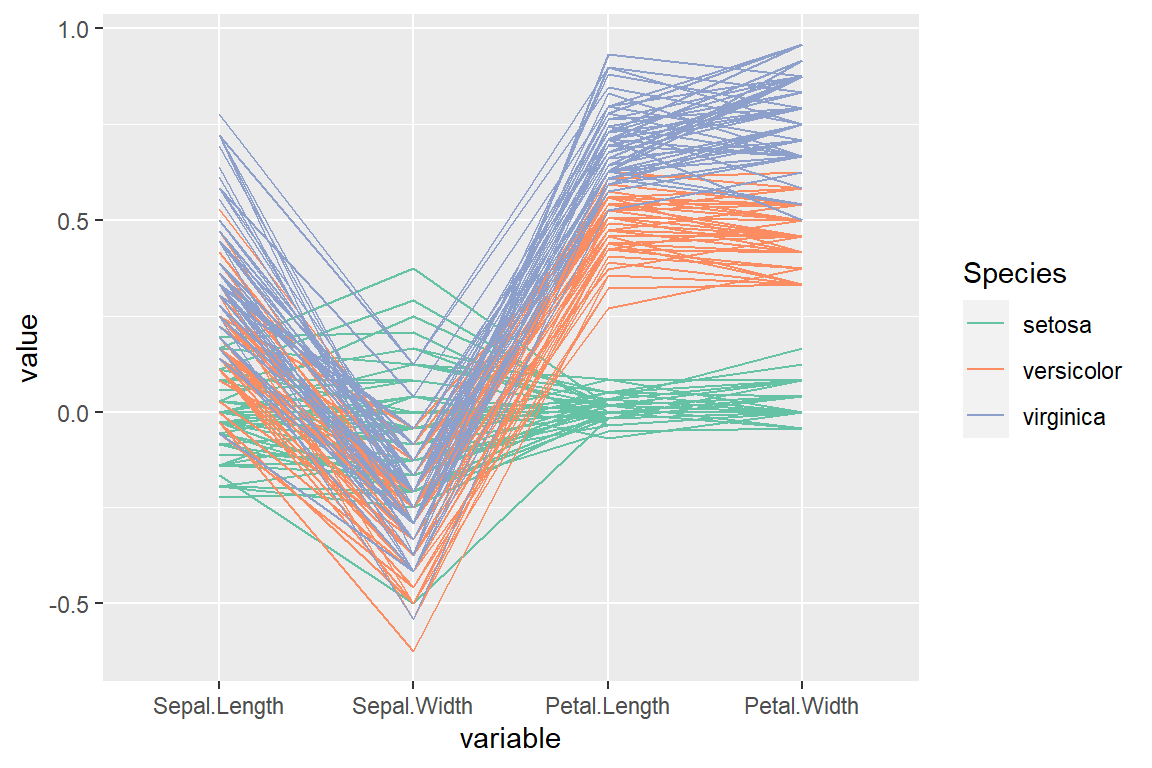## Ordering methods

There are also several methods for ordering the variables displayed on the X-axis. The default ordering is based on the selected columns, but you can also specify a custom order vector or select one of the methods displayed below. Type `?ggparcoord` for further details for each mehtod.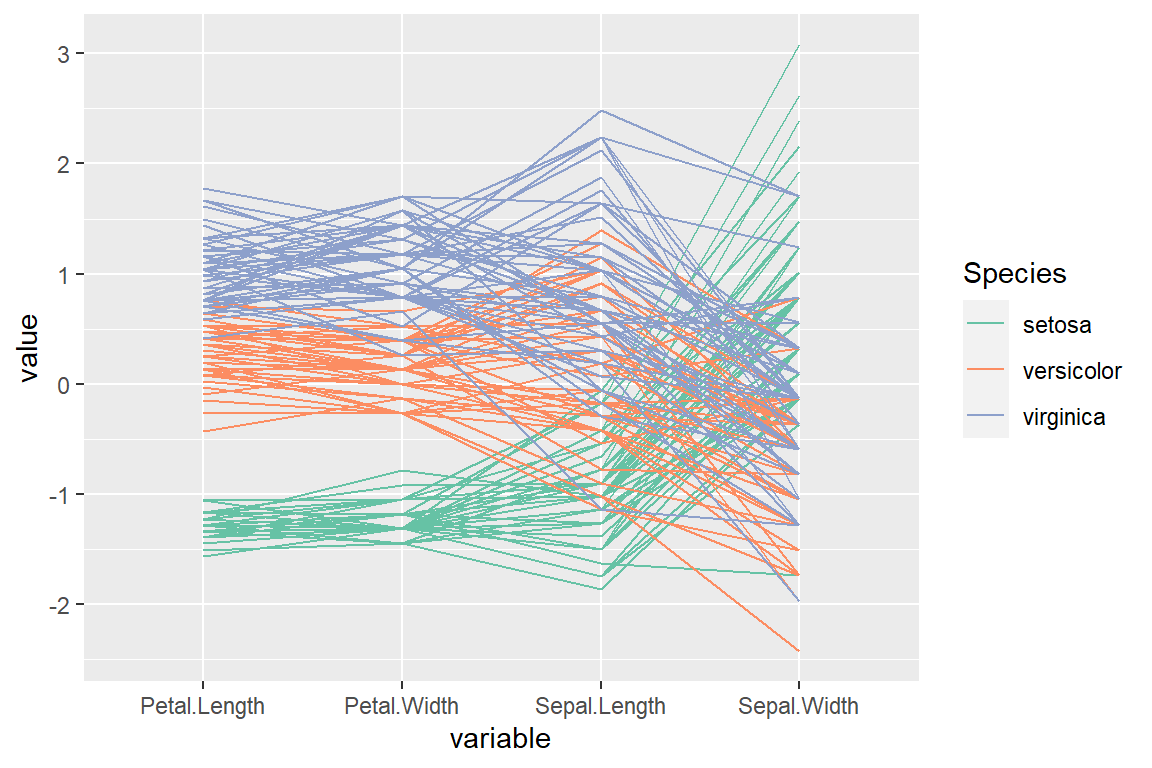“anyClass” order (by their maximum of k F-statistics)

``````# install.packages("GGally")
library(GGally)

ggparcoord(data = iris,
columns = 1:4,
groupColumn = "Species",
order = "anyClass") +
scale_color_brewer(palette = "Set2")``````“allClass” order (by their F-statistic from an ANOVA)

``````# install.packages("GGally")
library(GGally)

ggparcoord(data = iris,
columns = 1:4,
groupColumn = "Species",
order = "allClass") +
scale_color_brewer(palette = "Set2")``````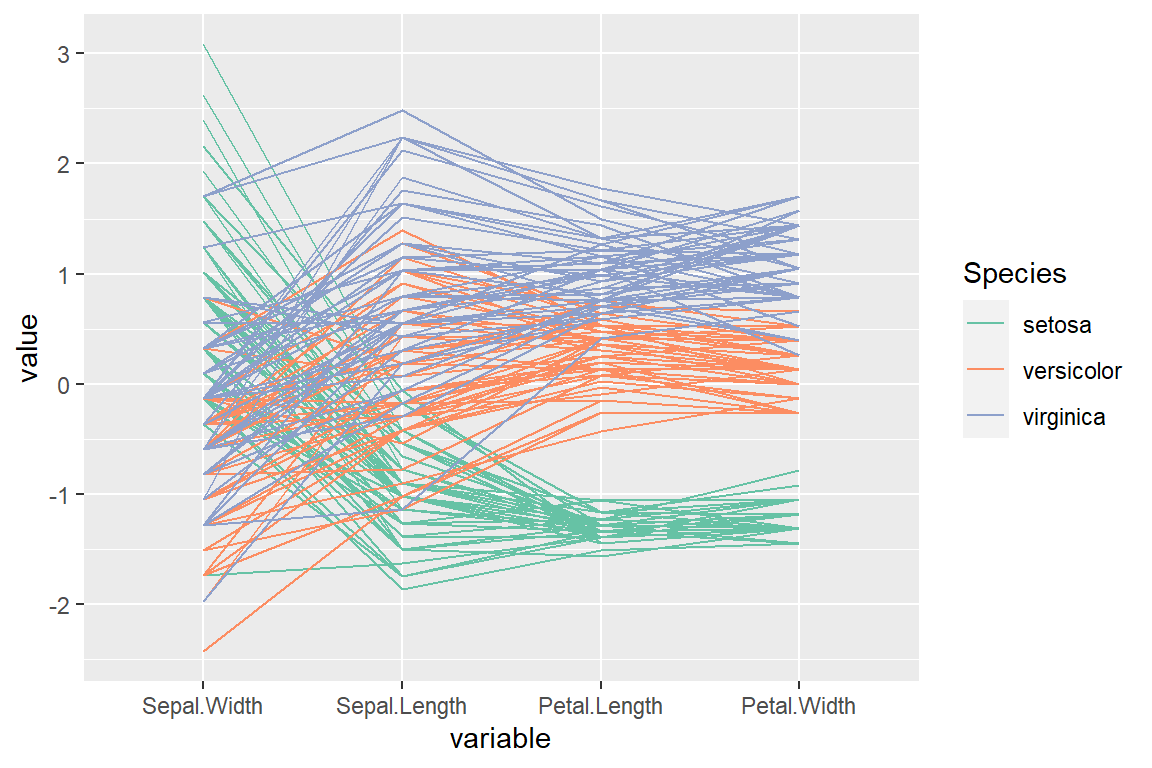“skewness” order (by their sample skewness)

``````# install.packages("GGally")
library(GGally)

ggparcoord(data = iris,
columns = 1:4,
groupColumn = "Species",
order = "skewness") +
scale_color_brewer(palette = "Set2")``````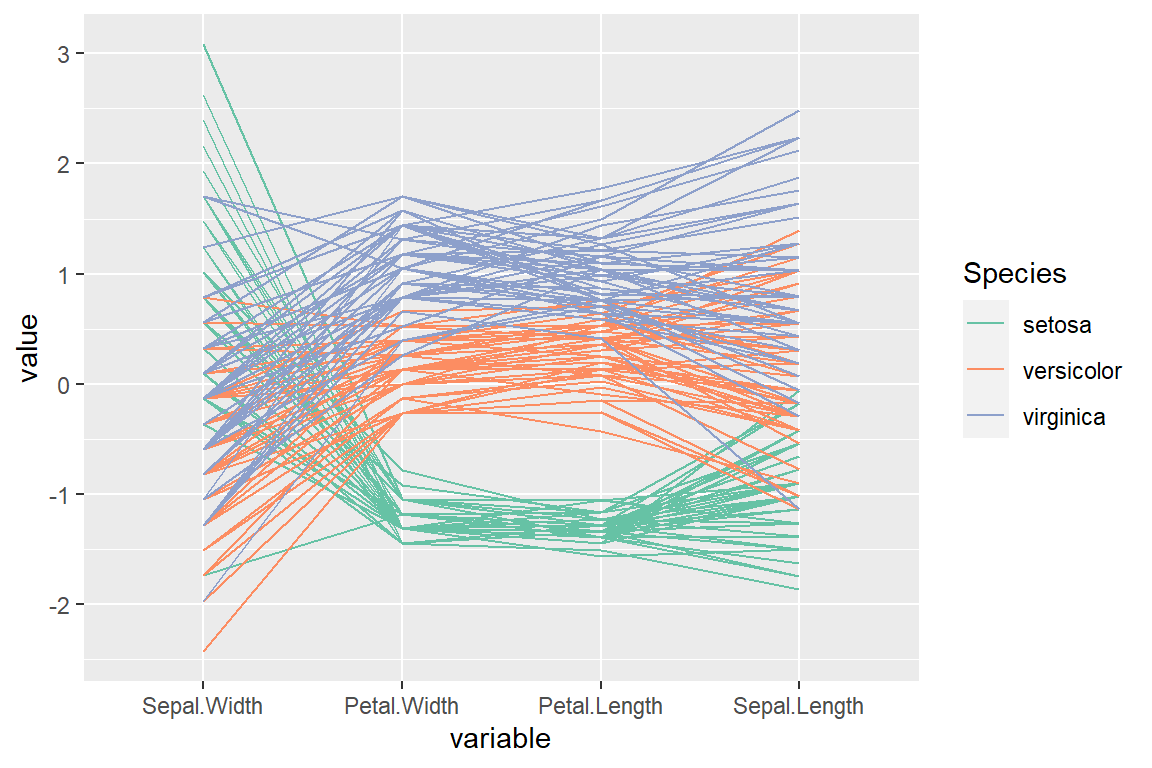“Outlying” order (by the scagnostic measure)

``````# install.packages("GGally")
# install.packages("scagnostics")
library(GGally)

ggparcoord(data = iris,
columns = 1:4,
groupColumn = "Species",
order = "Outlying") +
scale_color_brewer(palette = "Set2")``````

## Parallel coordinates for each group

You can make use of the `facet_wrap` function to create a parallel coordinates plot for each of the groups.

``````# install.packages("GGally")
library(GGally)

ggparcoord(data = iris,
columns = 1:4,
alphaLines = 0.2,
boxplot = TRUE,
groupColumn = "Species",
order = "Outlying") +
scale_color_brewer(palette = "Set2") +
facet_wrap(~ Species)``````